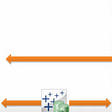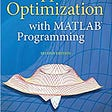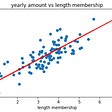# Vector

`import numpy as npVr = np.array([[1, 2, 3]]) # row vectorVc = np.array([,, ]) # Column vector## To find out the shape of a vector Vr.shape # output => (1, 3)Vc.shape # output => (3, 1)`

# Vector Operations

## 1 . Addition of vectors

`x = np.ones(3)       # Vector of three onesy = np.array((5, 4, 6))   # Converts tuple (5, 4, 6) into arrayx + y                  #output => array([8, 7, 9])#Similarly, subtraction of two vectorsy - x                 # output => array([2, 1, 3]) `

## 2. Scalar Multiplication of Vector

`4 * x     # output => array([4, 4, 4])5 * y     # output => array([ 25, 20, 30])`

## 3. Dot Product

`X = np.array([1,2,3])Y = np.array([4,5,6])np.dot(x,y)                 # output= 32`

`X = np.array([2, 3, 4])Y = np.array([5,6, 7])H = X * Y           # 2 *5, 3* 6, 4*7print(H)             #output = [10, 18, 28]`

# Matrices

`M = np.array([[1, 2], [3, 7], [-1, 5]])# A 3 row * 2 column matrix`

## Matrix multiplication

`A = np.array([[1, 7], [2, 4]])B = np.array([[3, 3], [5, 2]])C = np.linalg.dot(A, B)print(C)#output [[38 17] [26 14]]`
`a = np.array([[2,3], [2,3]])b = np.array([[3,4], [5,6]])# Uses python's multiply operatorprint(a * b) #output [[ 6, 12], [10, 18]]`

## Power Matrix

`from numpy.linalg import matrix_powerM = np.array([[1, 4], [5, 2]])print(M)           M2 = matrix_power(M, 2)        # M*M or M to the power 2print(M2)                 # [[21, 12], [15, 24]]`

## Transpose

`A = np.array([[1, 2],[3,4],[5, 6]])AT = A.transpose()  #Give the transpose#shorter notationA.T                 #Give the transpose`

## Determinant

`a = np.array([[1, 2], [3,4]])dtr = np.linalg.det(a)        # 1*4 - 2*3 print(dtr)                   # output = -2.0`

## Inverse

`C = np.array([[1,3],[4,5]])C_inverse = np.linalg.inv(C)print(C_inverse)#code end                 #output =>[[-0.71428571  0.42857143] [ 0.57142857 -0.14285714]]`

# Other Ways to create Matrices and Vectors

`V = np.zeros((2,2))             #Creates an array of all zeroesprint(V)X = np.ones((2,3))              # Creates an array of all onesprint(X)Y = np.full((2,4), 7)   # Creates an array of constant number 7print(Y)Z = np.eye(3)          #Creates an identity matrix of 3 x 3 print(Z)R = np.random.random((2,2))#Creates an random array of dimension 2x2print(R)`

# Performing Linear Regression with Linear Algebra

## Python code

`import numpy as np                   # linear algebraimport pandas as pd                  # import matplot.pyplot as plt         # graphsX = np.array([[1, 49],              [1, 69],              [1, 89],              [1, 99],              [1, 109]])Y = np.array([,              ,              ,              ,              ])`
`XT = X.transpose()a = np.dot(XT, X)`
`b = np.linalg.inv(a)`
`c = np.dot(XT, Y)`
`A = np.dot(b,c)`
`slope= round(float(A), 2)              `
`y_intercept = round(float(A, 2)`
`X = np.array([, , , , ]) #Column matrix of Xdf = pd.Dataframe(data=X, columns=["X"])print(df)          #prints our X element in a tabledf["Y"] = Yfx = X*slope + 211df["fx"] = fx##difference between the actual Y and predicted Y_i = Fxdf['E_i'] = df["Y"]- df["fx"]`
`E_i = [[x] for x in df["E_i"]]             # List comprehension E_i = np.array(E_i)ET = E_i.transpose()SSE = np.dot(ET, E_i)`
`N = 5                               # Number of data pointsMean_square_error = SSE/N`
`fig = plt.plot(df["X"], df["Y"])fig.show()`
`fig = plt.plot(df["X"], df["fx"])`
`m, b = np. polyfit(df["X"], df["Y"], 1)`
`plt.plot(df["X"], m*df["X"] + b)`

--

--

--

## More from Pratik Sharma

Learner | Developer |

Love podcasts or audiobooks? Learn on the go with our new app.

## Launching a VPC network for deploying WordPress with Database on AWS using Terraform## How to Install MongoDB## AirGap takes the non-custodial test## What are Hyper Files in Tableau## Meet TestFlight on Mac WWDC 2021## Pratik Sharma

Learner | Developer |

## NumPy — Mathematical Computing with Python## Exploring Correlation in Python## Machine Learning: Simple Linear Regression With Python## The most Important Libraries for Machine Learning Beginner .